## An amoeba has 1.00 x 1016 protons and a net charge of 0.300 pC. Assuming there are 1.88 x 106 fewer electrons than protons, If you paired th

Question

An amoeba has 1.00 x 1016 protons and a net charge of 0.300 pC. Assuming there are 1.88 x 106 fewer electrons than protons, If you paired them up, what fraction of the protons would have no electrons

in progress 0
6 months 2021-07-14T06:49:17+00:00 1 Answers 26 views 0

## Answers ( )

The fraction of the protons would have no electrons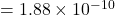Explanation:

We are given that

Amoeba has total number of protons=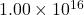Net charge, Q=0.300pC

Electrons are fewer than protons=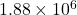We have to find the fraction of protons would have no electrons.

The fraction of the protons would have no electrons

=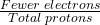The fraction of the protons would have no electrons

=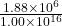Hence, the fraction of the protons would have no electrons